# median blur

(December 2010)

## median

The median average of a set of numbers is the number in the middle, so that half of the set is lesser than (or equal to) the median.

To calculate the median of a list, sort the list and pick the number in the middle.

 1 1 2 3 4 5 99 ⇧

The median of a list with an even number of elements is the average of the two middle elements.

## 2D median blur

Consider an input image with noise added:

```    img[:, :] = 100
for y in range(HEIGHT):
for x in range(WIDTH):
if random.randint(0,100) < percent:
img[y][x] = random.randint(0,255)
```

For every pixel in the output image, we take the corresponding pixel from the input image, and all surrounding pixels within a specified radius, and calculate the median of their values.

Here's what this looks like for different amounts of noise:

Noise Input Output Radius
10%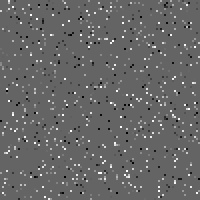3px
50%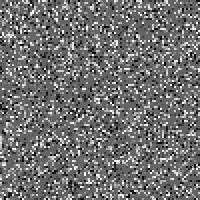3px

Note that the median is statistically robust as long as 50% of your data isn't noise. Once the majority of your samples are wrong, your outputs will also be wrong: you can see a few flecks above where we got unlucky with random clustering.

Here's what median blur of white noise looks like at different radii:

Noise Input Output Radius
100%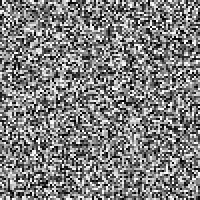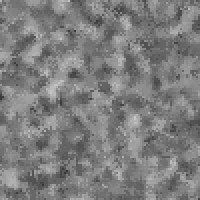3px
100%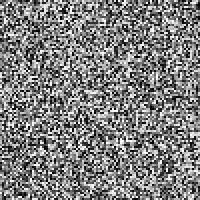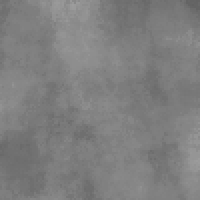10px

## noise reduction in photos

Here are crops of two (out of a series of three) photos of the same scene:The images are aligned (registered) but have differing noise. This is the normalized difference between them: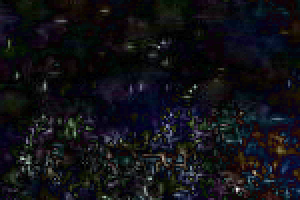Stack the images up, and for every pixel, take the average of the matching pixel in each source image:

 mean median2D median blur of the first image alone, compared to a median blur that uses data from all three images:

 1 image 3 images